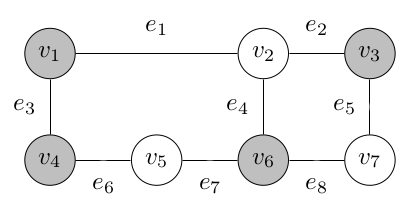# Proving the set-covering problem is NP-complete (using reduction from the vertex cover problem)

In this post, we will prove that the decision version of the set-covering problem is NP-complete, using a reduction from the vertex covering problem (which is NP-complete). This is Exercise 35.3-2 in CLRS3.

We will follow the template given in an earlier post.

## Problem Statement

We seek to answer whether a set cover $$\mathcal{C}$$ exists for $$X$$ (the set containing the elements to be covered), containing a maximum of $$k$$ sets.

## Showing the problem is in NP

Let $$\mathcal{C} = \{S_1, S_2, \ldots, S_n\}$$ be the certificate. We assume that each $$S \in \mathcal{C}$$ uniquely covers at least one $$x \in X$$, so:

\begin{aligned} |\mathcal{C}| &\leq |X|\\ \forall S \in \mathcal{C} : |S| &\leq |X| \end{aligned}

This gives us that we can verify that $$n \leq k$$ and that $$\mathcal{C}$$ covers $$X$$ in $$\mathcal{O}(|X|^2)$$, which proves the problem is in NP.

## Showing the problem is NP-hard

We will show that the decision version of the set-covering problem is NP-hard by showing:

$\text{Vertex-Cover} \leq_\text{P} \text{Set-Cover}$

### Reduction

A vertex cover of an undirected graph $$G = (V, E)$$ is a subset $$V' \subseteq V$$, such that each $$e \in E$$ is adjacent to at least one $$v' \in V$$. Its decision version is to determine whether a vertex cover of at most size $$k$$ exists for $$G$$.

We can reduce any instance of decision-vertex-cover for $$G = (V, E)$$ to an instance of decision-set-cover by letting $$X = E$$ and letting the family of sets $$\mathcal{F} = {S_v | v \in V\ \land \text{deg}(v) > 0}$$, such that $$S_v$$ contains all edges in $$E$$ adjacent to $$v$$. We can clearly perform this reduction in $$\mathcal{O}(|E| + |V|)$$, which is in polynomial time.

We will now prove for any vertex cover $$V'$$ for $$G$$ and a set cover $$\mathcal{C}$$ for sets $$X$$ and $$\mathcal{F}$$ constructed in accordance with the reduction above:

$\exists V'\subseteq V : |V'| \leq k \iff \exists \mathcal{C} \subseteq \mathcal{F} : |\mathcal{C}| \leq k$

Since $$X = E$$, and we know $$V'$$ is a vertex cover for $$G = (V, E)$$, we can find $$\mathcal{C}$$ for $$(X, \mathcal{F})$$ by selecting from $$\mathcal{F}$$ all sets corresponding to $$v' \in V'$$.

Similarily, if $$\mathcal{C}$$ exists, by the way we defined the reduction, we know it covers $$X = E$$. This means We can find $$V'$$ by taking the vertices corresponding to $$S_v \in \mathcal{C}$$.

Due to the 1:1 correspondence of vertices in $$V'$$ and sets in $$\mathcal{C}$$: $$|V'| = |\mathcal{C}|~\blacksquare$$

### Example

An example is probably useful here. See the image below for an example of a vertex cover of size 4 (with shaded nodes being in $$V'$$).The result of performing the reduction for this example is:

\begin{aligned} \mathcal{F} = \{S_{v_1} &= \{e_1, e_3\}, \\ S_{v_3} &= \{e_2, e_5\},\\ S_{v_4} &= \{e_3, e_6\},\\ S_{v_6} &= \{e_4, e_7, e_8\}\} \end{aligned}